# Discussing Proof of Special Relativity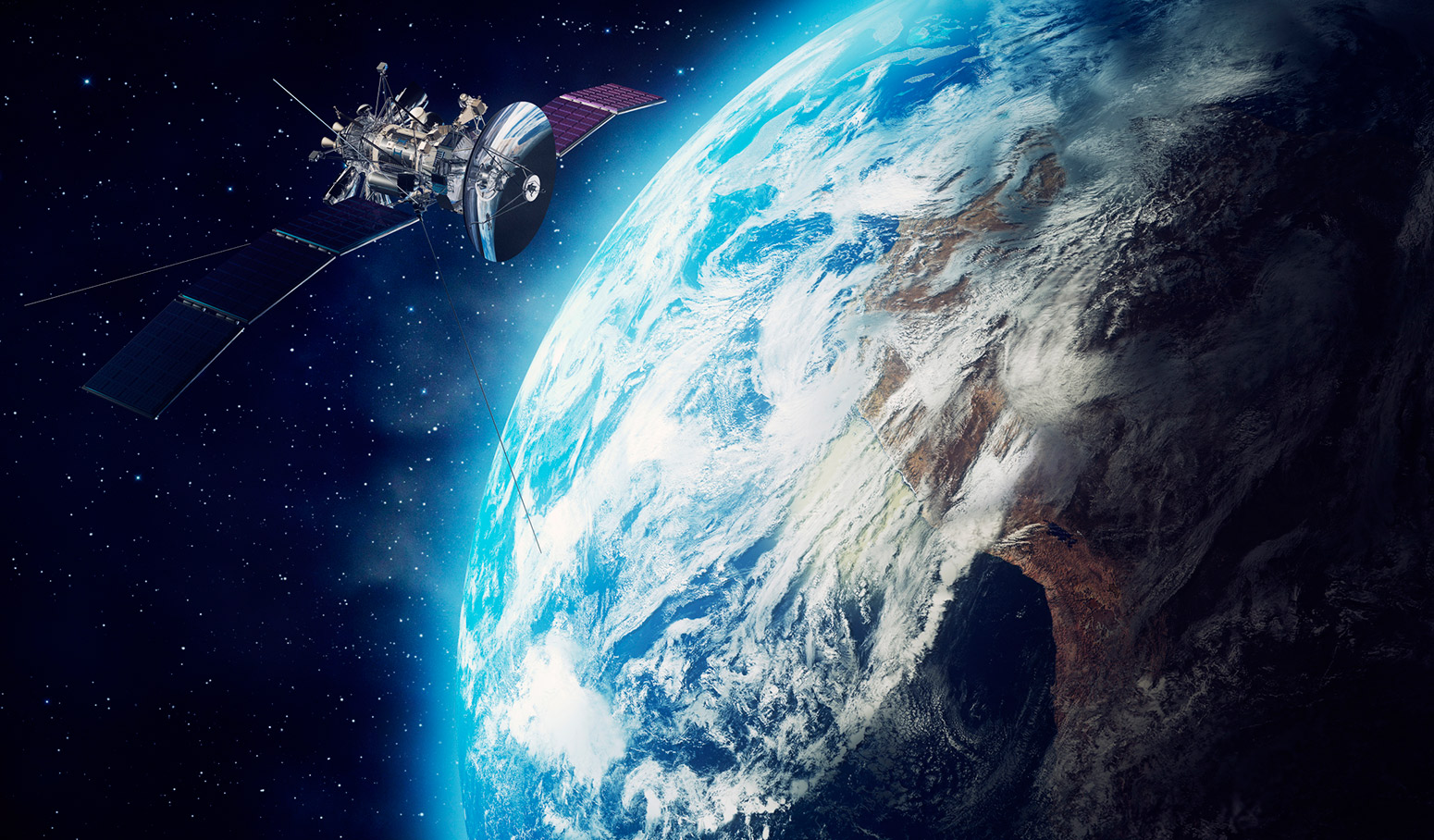We’re going to pick the Lorentz equations back up in a later entry. I feel like it’s worth it to try to tackle the mathematical reasoning behind them thoroughly and objectively so we can either gain enough insight into them to verify its reasoning or to completely discard them as fantasy.

For now, I will say that further research reveals that sources-stated origination claims of the light-like separated events equation necessarily follow my reasoning in its origination. I can get the equation to a basic Pythagorean equation, but sources that I’ve read since them have stated that this is not the case.

Let’s take a look at the problem on the other side, however. Over the last few decades, we’ve seen the science community present things like GPS satellites as evidence or proof of the effect of Lorenz’s equations. For simplicity’s sake, we will state the following hand-wave exercise to get from the light-like separated events equation to get to the Lorentz Transforms. We start by the light-like separated events equation: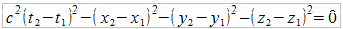This is the equation for one event. By this rational, we can pick another event anywhere else in the known universe and end up with the same equation, but with different points in space and time: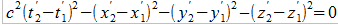Because both equations equal zero, they also are equal to each other, so we can write the following: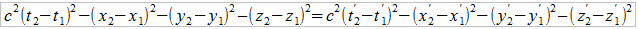We can discuss the implecations of 0=0 in a subsequent blog, but for now let’s just acknowledged that what we’re seeing here is the sum of the components equaling zero, but the components themselves being different accounting for the need of such a formulation.

This is where we end up hand-waving all the simplifications and abstractions and simply state that we move our frames so that one event is not moving in relation to the other and that all translation is occurring along the x-axis. The resulting equations are the Lorentz Transforms: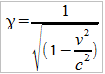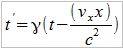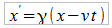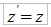The above equations are the Lorentz transform. They imply a concept called time-dilation. Simply put, the faster an object is traveling through space-time, the slower time will progress for that object. This is implied through the velocity component of the second equation of the Lorenz transform.

Since the latter half of the 20th century, people have been pointing to atomic clocks on fast-moving airplanes and Global Positioning Satellites as evidence or proof of this time-dilation property.

In October 1971, Hafele and Keating flew cesium-beam atomic clocks, initially synchronized with the atomic clock at the US Naval Observatory in Washington, D.C., around the world both eastward and westward. After each flight, they compared the time on the clocks in the aircraft to the time on the clock at the Observatory. Their experimental data agreed within error to the predicted effects of time dilation. Of course, the effects were quite small since the planes were flying nowhere near the speed of light.”

To achieve this level of precision, the clock ticks from the GPS satellites must be known to an accuracy of 20-30 nanoseconds. However, because the satellites are constantly moving relative to observers on the Earth, effects predicted by the Special and General theories of Relativity must be taken into account to achieve the desired 20-30 nanosecond accuracy.

Because an observer on the ground sees the satellites in motion relative to them, Special Relativity predicts that we should see their clocks ticking more slowly (see the Special Relativity lecture). Special Relativity predicts that the on-board atomic clocks on the satellites should fall behind clocks on the ground by about 7 microseconds per day because of the slower ticking rate due to the time dilation effect of their relative motion .

Further, the satellites are in orbits high above the Earth, where the curvature of spacetime due to the Earth’s mass is less than it is at the Earth’s surface. A prediction of General Relativity is that clocks closer to a massive object will seem to tick more slowly than those located further away (see the Black Holes lecture). As such, when viewed from the surface of the Earth, the clocks on the satellites appear to be ticking faster than identical clocks on the ground. A calculation using General Relativity predicts that the clocks in each GPS satellite should get ahead of ground-based clocks by 45 microseconds per day.”

http://www.astronomy.ohio-state.edu/~pogge/Ast162/Unit5/gps.html

To summarize, the results of the readings of atomic clocks correlate with expected results predicted both by the Lorentz transforms and also Einstein’s General Relativity as well.

Those of you who are skeptical-minded should be picking up on something right about now. The evidence correlates with the predictions made by the equations. It doesn’t necessarily prove anything, and you can inject skepticism into the evidence by simply reciting a well-known logical argument here:

Correlation does not imply causation.

With this in mind, let’s mull over a few facts when considering the whole problem of atomic clock accuracy in relation to its relative speed.

1. GPS satellites travel around the earth at 14,000km/hr
2. GPS satellites travel around the earth about 20,000km above the surface of the earth.

http://www.astronomy.ohio-state.edu/~pogge/Ast162/Unit5/gps.html

The GPS satellites do, indeed, travel above the earth at a very fast speed. But it’s all relative, right? Here are some more facts:

1. The Earth travels around the sun at nearly 30km/s. That’s 108,000km/hr.
2. The entire solar system rotates around our galaxy at a speed of 792,000km/hr.
3. Even worse, our galaxy travels in space at around 3,600,000km/hr.

https://www.scientificamerican.com/article/how-fast-is-the-earth-mov/

Also let’s consider that the speed of light is considered an absolute no matter what frame of reference that you are traveling in. By the postulates of relativity, you cannot make a beam of light travel any slower or faster no matter what speed you are traveling in the universe.

So if there’s an absolute on the speed of light in the known universe, then you can also logically deduce that there must be an absolute zero in terms of speed. If there’s an absolute zero in terms of speed and an absolute maximum, and the Lorentz equation predicts time-dilation based on an objects speed in relation to the speed of light, then time dilation is based on something absolute.

Given then, the stated facts of the motions of our earth, our solar system, and our galaxy, how is it that we can state that corrections to GPS satellites we make due to the effects we observe is proof of the truthfulness of the Lorentz Transforms?

You would think that the motions of our celestial bodies through space, coupled with the fact that we do experience a rotation of earth, an orbital rotation about the sun, and galactic rotation about the Milky-way’s center would produce a daunting rat’s-nest of complex variables that GPS satellites would have to constantly correct for. I highly doubt that GPS satellites have the computational power to keep track of all these variables, and I haven’t read that engineers in charge of the satellites even took influences outside of speed in relation to earth.

This is not to say that the equations haven’t proved to be useful We see, from the ohio-state post that such calculations have been used to build the GPS system based on some of the calculations we get from Special Relativity and General Relativity. We can use those results to engineer fantastic things like GPS but still leave room to question whether or not Relativity is right or wrong.

After all, most of our mechanical engineering that we use today still rely on physics developed by Sir Isaac Newton. And we still use his equations today for the majority of our engineering calculations even though we have stated that general relativity is the more correct understanding of the universe over his.

So maybe it’s correct to state that we can use Special Relativity and General Relativity as a model of something that we still have yet to understand. This leaves room to give Einstein credit where it’s due, yet also admit to ourselves that we still have much to learn about this universe.